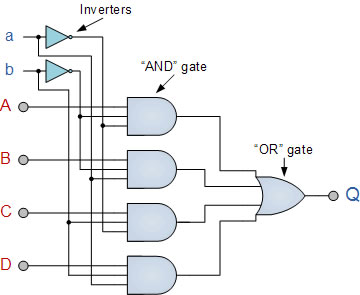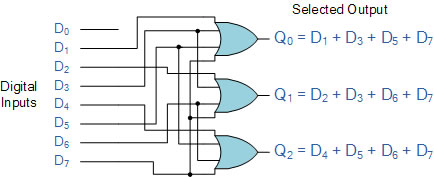# Difference between Multiplexer and Encoder

##### Key Difference: A multiplexer or MUX is a combination circuit that contains more than one input line, one output line and more than one selection line. Whereas, an encoder is also considered a type of  multiplexer but without a single output line. It is a combinational logic function that has 2^n (or fewer) input lines and n output lines.

Multiplexer and encoder both are very important for the digital electronics. They are types of combinational logic circuits. The difference between them may be due to some confusion, as encoder is also considered to be a kind of multiplexer. Thus, it is important to find out the characteristics of both which will help in differentiating between them.

To understand the multiplexer and encoders, the concept of combinational circuits must be clear. Combinational logic circuits are defined by the logical function of their current input state. The state can be either 0 or 1, at any instance. In a combinational logic circuit,  the output is dependent on the combination of its inputs. If in any case the change in a state of input occurs, then the output is also affected by the change. These circuits are basically made up from three basic gates:

1. AND
2. OR
3. NOT

These gates are combined to produce simple or complicated switching circuits. The function of the combinational logic circuit can easily be obtained by implementation of Boolean algebra, truth table or by logic diagrams. These combinational logic circuits are classified into three broad categories:

1. Arithmetic and logical functions
2. Data Transmission
3. Code Converters

The data transmissions category includes the multiplexers, demultiplexers, encoders and decoders. Thus, this particular category is of great importance in terms of defining the differences between the multiplexer and encoder.A multiplexer is also known as a data selector, it consists of a group of data inputs and a group of control inputs. It selects one of many data lines and outputs that into a single line. A multiplexer with n control inputs have 2^n data inputs and 1 output. This digital switch is also known as MUX.

Multiplexers are also used in communications; the telephone network can be understood as very large virtual multiplexer that consists of many smaller discrete ones. It is not possible to have a direct link between one phone and the other, therefore, the network uses multiplexing and converts individual telephone signals onto one of a small number of wires as calls are placed. At the receiving end, demultiplexer is present and that chooses the right destination among many.

An encoder is also a kind of combinational circuit that is used to convert binaryinformation from 2^n inputs to n outputs. The encoder is used for purposes like standardization, speed, secrecy, security, or saving space by shrinking size. When a bit comes in on an input wire, the encoder outputs the physical address of that wire. It takes 2^n inputs and gives out n outputs; the enable pin should be kept 1 for enabling the circuit. Examples of Encoders are following:-

Priority encoders

Decimal to BCD encoder

Octal to binary encoder

An encoder is a device that can be used to change a signal (such as a bitstream) or data into a specific code. The code may be used for any purpose like, for the compression of information required for transmission and storage, for encryption or for adding redundancies to the input code.

Thus, we can conclude that they both are important for digital electronics and thus both are used in various components. They both have inputs and outputs. They differ in the number of inputs and outputs. The multiplexer can be defined as a converter of 2^n inputs to 1 output, whereas the encoder coverts 2^n inputs into n outputs.

Images Courtesy: electronics-tutorials.ws